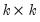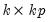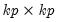User’s Guide : Multiple Equation Analysis : Vector Autoregression (VAR) Models : Data Members of a VAR

Data Members of a VAR
By their nature, estimated VARs contain a large number of parameters and results. Views and procs can help you to visualize and interpret results, but there are times when you need to access the parameters of the VAR directly, in order to display results in an alternative form, or to perform supplementary computation.
Data members are available to provide you access to the results of the VAR. We focus here on a few of the more commonly used members, but many others are available (see “Var Data Members” a complete list of data members that are available for a VAR object).
Coefficients
Coefficients of (the unrestricted) VARs may be accessed by referring to the elements of a two dimensional array “C”. The first dimension of C refers to the equation number of the VAR, while the second dimension refers to the variable number in each equation.
For example, “C(2, 3)” is the coefficient of the third regressor in the second equation of the VAR. The C(2, 3) coefficient of a VAR named VAR01 can then be accessed by the command
scalar c23 = var01.c(2,3)
To access the entire matrix of coefficient results (in the order they are displayed in the estimation output), you may use the @coefmat member:
matrix mycoefs = var01.@coefmat
To view the correspondence between the elements of C and the variables and estimated coefficients, select View/Representations from the VAR toolbar.
Lag Coefficients
Any dynamic analysis of a VAR requires knowledge of \ the lag coefficients of the model. EViews offers several data members that return the lag coefficients in various forms.
Thematrix of lag coefficients for a given lag may be obtained using the @lagcoef(k) data member. Rows and columns of the matrix will correspond to the endogenous variables in the VAR, in the order in which they are specified. For example
matrix lagcoef3 = var01.@lagcoef(3)
will give the coefficients of the third lag of the endogenous variables. Note that if a given lag is excluded from the VAR specification, the result will be a matrix of 0s.
Similarly, the @lagcoefs member will provide you with thematrix containing the full set of horizontally concatenated lag coefficient matrices,
matrix lagcoef_all= var01.@lagcoefs
If you require thecompanion form of the lag coefficient matrix, you may use the @companion data member:
matrix companion = var01.@companion
To obtain thesum of the lag coefficient matrices, use the @lagcoefsum data member:
matrix lagcoef_sum = var01.@lagcoefsum